Next: Rotation Up: Vectors and Vector Fields Previous: Vector Area

# Vector Product

We have discovered how to construct a scalar from the components of two general vectors,and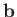. Can we also construct a vector that is not just a linear combination ofand? Consider the following definition: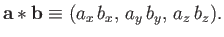(A.36)

Is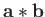a proper vector? Suppose that,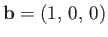. In this case,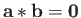. However, if we rotate the coordinate axes through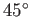aboutthen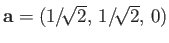,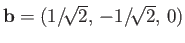, and. Thus,does not transform like a vector, because its magnitude depends on the choice of axes. So, previous definition is a bad one.

Consider, now, the cross product or vector product:(A.37)

Does this rather unlikely combination transform like a vector? Let us try rotating the coordinate axes through an angleaboutusing Equations (A.20)-(A.22). In the new coordinate system,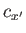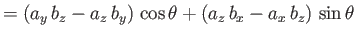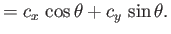(A.38)

Thus, the-component oftransforms correctly. It can easily be shown that the other components transform correctly as well, and that all components also transform correctly under rotation aboutand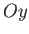. Thus,is a proper vector. Incidentally,is the only simple combination of the components of two vectors that transforms like a vector (which is non-coplanar with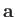and). The cross product is anti-commutative,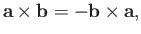(A.39)

distributive,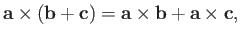(A.40)

but is not associative,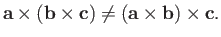(A.41)

The cross product transforms like a vector, which means that it must have a well-defined direction and magnitude. We can show thatis perpendicular to bothand. Consider. If this is zero then the cross product must be perpendicular to. Now,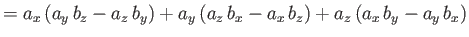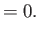(A.42)

Therefore,is perpendicular to. Likewise, it can be demonstrated thatis perpendicular to. The vectors,, andform a right-handed set, like the unit vectors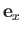,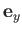, and. In fact,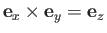. This defines a unique direction for, which is obtained from a right-hand rule. (See Figure A.8.)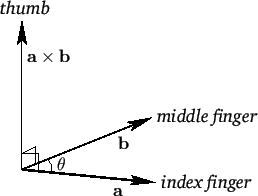Let us now evaluate the magnitude of. We have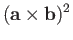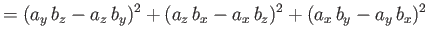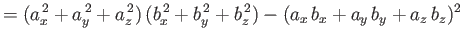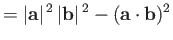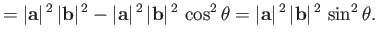(A.43)

Thus,(A.44)

whereis the angle subtended betweenand. Clearly,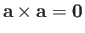for any vector, becauseis always zero in this case. Also, if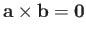then either,, oris parallel (or antiparallel) to.

Consider the parallelogram defined by the vectorsand. (See Figure A.9.) The scalar area of the parallelogram is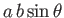. By convention, the vector area has the magnitude of the scalar area, and is normal to the plane of the parallelogram, in the sense obtained from a right-hand circulation rule by rotatingon to(through an acute angle). In other words, if the fingers of the right-hand circulate in the direction of rotation then the thumb of the right-hand indicates the direction of the vector area. So, the vector area is coming out of the page in Figure A.9. It follows that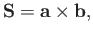(A.45)Suppose that a force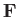is applied at position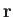, as illustrated in Figure A.10. The torque about the originis the product of the magnitude of the force and the length of the lever arm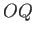. Thus, the magnitude of the torque is. The direction of the torque is conventionally defined as the direction of the axis throughabout which the force tries to rotate objects, in the sense determined by a right-hand circulation rule. Hence, the torque is out of the page in Figure A.10. It follows that the vector torque is given by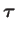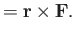(A.46)The angular momentum,, of a particle of linear momentumand position vectoris simply defined as the moment of its momentum about the origin: that is,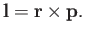(A.47)Next: Rotation Up: Vectors and Vector Fields Previous: Vector Area
Richard Fitzpatrick 2016-03-31